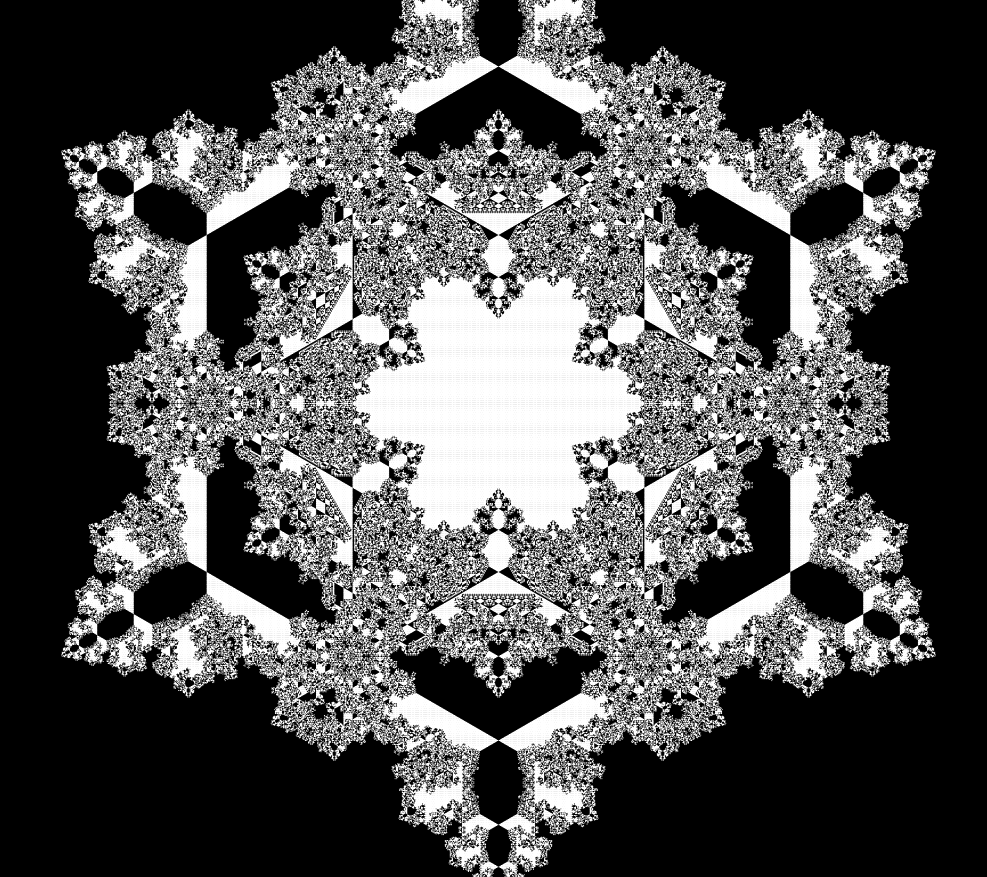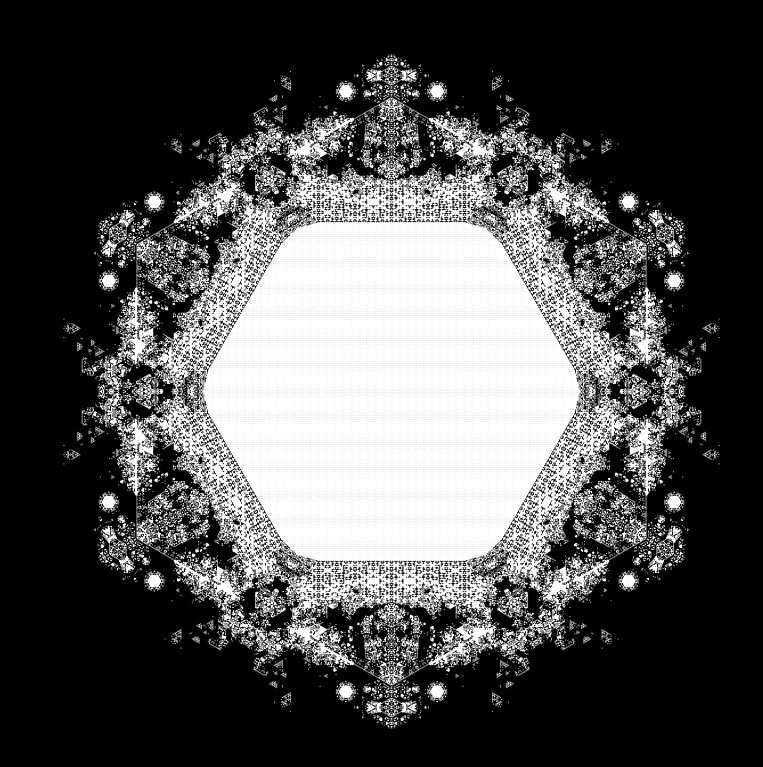## Friday, July 18, 2014

### Symmetric binary fractals

In a previous post I discussed fractal automata, which are like cellular automata (e.g. Conway's game of life) but include larger and smaller scales as neighbours as well as nearby locations. This is summarised here: https://sites.google.com/site/tomloweprojects/scale-symmetry/automataFinder

A subset of these systems are static fractals, generated by applying binary rules to lower resolution maps.
It is useful to try and find a setup with as much symmetry as possible and with as few degrees of freedom as possible. The default system operates on a 2d grid and so exhibits square symmetry. The 'type 7' fractals displayed in the above link use a semi-cheat to allow for octagonal symmetry. It works very well but has some drawbacks; it cannot be animated and isn't really octagonal in its symmetry, just close. It also doesn't extend to 3d which up to now has just cubic symmetry.

A new idea, which is the topic of this post, is to use extra dimensions to provide extra symmetry. If I use a 3d grid, and apply binary rules to convert low resolution voxels progressively to higher resolution ones, doubling the resolution each step, then take a long-diagonal cross section (the plane defined by x+y+z = 0) then the resulting 2d image has hexagonal symmetry. If I make the low resolution data just a 2x2x2 block of set voxels then the rules result in images such as these:Of course, one can easily make a grid with hexagonal symmetry (a triangular lattice), but the problem is that we need to elegantly register each cell to its lower resolution cell on a coarser version of the grid. For triangular lattices the obvious choice is for 4 triangles to refer to one parent triangle, however these triangles have different geometric locations relative to their parent, it can be the centre triangle or one of the three corner triangles, consequently you need different rules for each case and the rule set becomes larger which defeats the goal of minimising degrees of freedom (the number of possible rules).

By contrast, for 3d voxels, each of 8 child voxels occupy an identical corner of the parent 2x2x2 scaled voxel in my implementation. The ruleset I chose for the above images is therefore quite succinct... I base it only on the seven closest parent voxels, which, assuming cubic symmetry, gives 40 possible configurations of these seven voxels. The ruleset provides an on/off of the child voxel for each of these combinations, therefore 2^40 possible rulesets.

I can reduce this large search space down further by enforcing bit symmetry, this specifies that on and off are arbitrary so if a particular low res shape produces a particular high res fractal, then the negative (on and off swapped) low res shape produces a negative of the fractal. This reduces the search space down to 2^20 rulesets.

A last improvement is to acknowledge that a ruleset and its negative are functionally no different if you have bit symmetry, so we can normalise by choosing the version which converts all seven parent voxels being off to an off state for the child. Resulting in 2^19 rulesets.

You can of course also view the 3d shape generated, but in 3d you miss the details behind the surface:

#### Optimisation

For the 2d images, we only need a single plane of high resolution voxels, and these trace back to a thin slice through the coarser resolution voxels. Therefore the processing required is much less than for viewing the 3d output and is almost definitely O(n^2) for resolution nxn unlike O(n^3) for the 3d case. This also means that the initial seeds are only needed in a thin slice of the 3d voxel grid.

#### Random seeds

I can also seed randomly, which gives a better overview of how each ruleset looks on arbitrary input:#### Extensions

There are clearly variations on the rulesets that I'm using, one could look at a larger neighbourhood, or remove bit symmetry etc, I could also change the scaling factor (if the parent to child scaling is 3 rather than 2 then I am sure one can reconstruct the Koch snowflake for instance), but more degrees of freedom almost always gives less symmetric and less interesting (more noisy) results.

However, this method has several advantages, it probably can be made to animate (fractal automata), and it can be extended to higher dimensions.
3d fractals could be generated which have what is probably octahedral symmetry rather than cubic symmetry, by using a 3d long-diagonal cross section of a 4d binary fractal.
I am also wondering whether one can make use of the highly symmetric D4 lattice in 4d to create even more symmetric 3d fractals... however I'm not sure how elegantly you can register one 24-cell to a parent larger 24-cell.

#### Simpler

I'll finish with some examples from a simpler system where I just look at the 4 closest parents. In this case I haven't used bit symmetry. You can sort of see there is more simplicity in the rules. The last image uses a random seed.